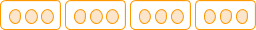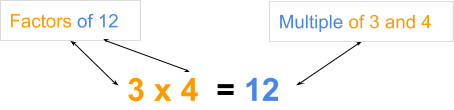# Factors

Go back to  'Factors, Multiples, Primes'

## A simple definition of factors

Factors of a given number are numbers that can perfectly divide that given number.

The word “factors” means part of. So, think of factors as a number part that when multiplied by a whole number gives you the orginal number. E.g. 3 is a factor of 12 because when 3 is multipied by a whole number it gives 12.

## Using Multiplication to understand Factors

Just like multiples, factors can also be understood using multiplication statements.

Example 2:

Let’s look at another example: 3 x 4 = 12

This tells us that we can obtain 12 by multiplying 3 by 4. So 12 is a multiple of 3 as well as 4.

At the same time, it tells us that 12 can be grouped or arranged in sets of 3 or 4.If a given number can be grouped in sets of another number, then that number is a factor of the given number.

So 3 and 4 are factors of 12.Factors and multiples are connected by the same multiplication statement. But this doesn’t give us all the factors of 12. Let’s see how to find all factors of a number.

### Using Division to understand Factors

Just as we used multiplication to understand multiples, we will use division to understand factors.

Example 3:

Let’s think of a number, say 12. Some numbers divide 12 perfectly, while other numbers do not. Dividing a number perfectly means not having any remainder. That is, the remainder should be zero.

E.g.     12 ÷ 6 = 2, with zero remainder. We say 6 divides 12 perfectly.

But,     12 ÷ 5 will give quotient 2 with remainder 2. We say 5 does not divide 12 perfectly.

Which numbers can perfectly divide 12? We can check number one by one…

 Finding all the factors of 12 The number we are checking Dividing 12 by that   number Does it divide 12 perfectly? 1 12 ÷ 1 = 12, remainder 0 Yes, so 1 is a factor of 12 2 12 ÷ 2 = 6, remainder 0 Yes, so 2 is a factor of 12 3 12 ÷ 3 = 4, remainder 0 Yes, so 3 is a factor of 12 4 12 ÷ 4 = 3, remainder 0 Yes, so 4 is a factor of 12 5 12 ÷ 5 give quotient 2 and remainder 2 No 6 12 ÷ 6 = 2, remainder 0 Yes, so 6 is a factor of 12 7 12 ÷ 7 give quotient 1 and remainder 5 No 8 12 ÷ 8 give quotient 1 and remainder 4 No 9 12 ÷ 9 give quotient 1 and remainder 3 No 10 12 ÷ 10 give quotient 1 and remainder 2 No 11 12 ÷ 11 give quotient 1 and remainder 1 No 12 12 ÷ 12 = 1, remainder 0 Yes, so 12 is a factor of 12 So the factors of 12 are: 1, 2, 3, 4, 6 and 12

Example 4:

Let’s consider another example. Can you find the factors of 8?

• Check which of the numbers 1, 2, 3, 4, 5, 6, 7 and 8 divide 6 perfectly.
• The numbers that give zero remainder are: 1, 2, 4 and 8
• So factors of 8 are 1, 2, 4 and 8.

Pro tip: Can you think of why we stopped checking at 8?

Only a number less than or equal to a given number can perfectly divide that number. Hence we stopped checking at 8 when finding factors of 8; and stopped at 12 when finding factors of 12 earlier.

The word “factors” means part of. So think of factors as a number part that when multiplied by a whole number gives you the original number. E.g. 3 is a factor of 12 because when 3 is multiplied by a whole number it gives 12.

### An activity to visualise factors

You can use Cuisenaire rods (or fraction rods) to visualise factors of a number.

Cuisenaire rods have different sizes and colours. The size determines the value of the rod.

Here are the steps to visualise the factors of 6.

Step 1: Arrange all Cuisenaire rods one next to the other so we know which colour/length denotes which number.

Step 2: Pick the rod that denotes 6. Lay it horizontally.

Step 3: Now we will see if 6 can be divided perfectly by other numbers. We start with 1. So pick up a few rods of size one. Stack them below the 6 rod. Do a few rods of 1 stack up to create the length 6? Yes, they do. So 1 is a factor of 6.

Step 4: Pick up a few rods of size 2. Do a few rods of 2 stack up to create the length 6? Yes, they do. So 2 is a factor of 6.

Step 5: Pick up a few rods of size 3. Do a few rods of 3 stack up to create the length 6? Yes, they do. So 3 is a factor of 6.

Step 7: Pick up a few rods of size 4. Do a few rods of 4 stack up to create the length 6? No. They either fall short or they exceed the length 6. So 4 is not a factor of 6.

Step 8: Pick up a few rods of size 5. Do a few rods of 5 stack up to create the length 6? No. They either fall short or they exceed the length 6. So 5 is not a factor of 6.

Step 9: Pick up a few rods of size 6. Does the rods of size 6 stack up to create the length 6? Yes, just one rod of size 6 is enough. So 6 is a factor of 6.

## Tips and Tricks to find Factors

• To find the first few multiples, simply remember the multiplication table. The values in the multiplication table are the first few multiples of the number.
• The difference between consecutive multiples of a number is equal to that number.
So if you’re given the 43rd multiple of a number and asked to find the 44th, simple add the number to the 43rd multiple. Similarly, subtracting the number from the 43rd multiple will give you the 42nd multiple.
E.g. 43rd multiple of 8 is 344.
So the 44th multiple of 8 will be 344 + 8 = 352.
And the 42nd multiple of 8 will be 344 - 8 = 336.
• Factors come in pairs such that when you multiply the two factors in the pair, they give you the number. This helps to make sure we’ve listed all factors
E.g. Factors of 24 in pairs are: 1 and 24, 2 and 12, 3 and 8, 4 and 6. Factors in a pair give 24 when multiplied.
Use this to find factors in pairs. As you start checking from 1, every time you find a factor you’ll also find the other factor in the pair using this pairing technique.
These pairs work for all numbers except perfect squares. Numbers like 25 will have the following pairs of factors: 1 and 25, 5 and 5.
• Use divisibility rules to quickly find the first few factors. Even numbers will always have 2 as a factor. If the digits of the number add up to a multiple of 3, then 3 is a factor. Numbers ending with 0 or
• A number that has an even number of factors, it cannot be a perfect square. A number that has odd number of factors, is a perfect square.
E.g. 16 has 5 factors (an odd number). So 16 is a perfect square (4 x 4 = 16)
18 has 6 factors (an even number). So 18 is not a perfect square.

### Common mistakes or misconceptions related to Factors and Multiples

• Misconception: The factors of a number are never ending (infinite).
Misconception: The multiples of a number are limited in number (finite)

Rote memorisation leads to confusion between properties of factors and multiples. Multiples of a number never end as you can keep adding the same number over and over. Factors are numbers that evenly divide a given number. They are finite.
• Misconception: 1 is a multiple of every number.
Misconception: 0 is a factor of every number

Once again, this misconception occurs due to rote memorisation. 1 divides every number perfectly. So it is a factor of every number. On the other hand, if you take any number and multiply it by 0, you obtain 0. So zero is a multiple of every number
• What are non-zero multiples of a number?
Because 0 is a multiple of each and every number, it doesn’t add too much new information to the situation. Hence it is ignored as a multiple. A technically correct way of ignoring zero as a multiple is to ask for non-zero multiples.
E.g. The first 5 multiples of 4 technically are: 0, 4, 8, 12 and 16. But if we are ignoring zero (As we often want to) we would say the first 5 non-zero multiples of 4 are 2, 8, 12, 16 and 20.
• Misconception: Non-zero multiples exclude all multiples that have the digit zero.
The first few non-zero multiples of 5 are 5, 10, 15 and 20. Notice that we include 10 and 20. Non-zero multiples exclude only the number 0 which is technically the very first multiple of 5. Multiples that have the digit zero, like 10 and 20, are not excluded.

1. List the first 5 multiples of 7.
______________________________

2. List all the factors of 30.
______________________________

3. Which number is a factor of every number?
______________________________

4. Which number is a multiple of every number?
______________________________

5. The 36th multiple of 7 is 252. What will the 37th multiple of 7 be?
______________________________